﻿ Multiplication Worksheets and Printouts

# Multiplication Facts, Multiplication Fluency Worksheets

## Multiplication Facts Worksheets, Extension of CCSS 2.OA.4, Operations and Algebraic Thinking

###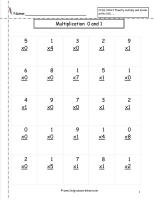Multiplication by zero and one Worksheet,  2

Common Core State Standards: Extension of CCSS 2.OA.4, CCSS 3.OA.7
Students multiply numbers by 0 and 1.

###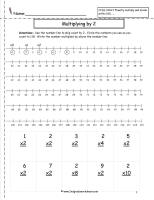Multiplication by Two with Numberline Worksheet

Common Core State Standards: Extension of CCSS 2.OA.4, CCSS 3.OA.7
Students multiply numbers by 2 with included numberline for skip counting.

###Multiplication by Two with Hundred Chart Worksheet

Common Core State Standards: Extension of CCSS 2.OA.4, CCSS 3.OA.7
Students multiply numbers by 2 with included hundred chart for skip counting

###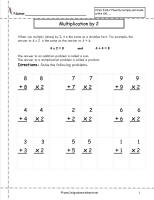Multiplication by Two with Doubles Worksheet

Common Core State Standards: Extension of CCSS 2.OA.4, CCSS 3.OA.7
Students multiply numbers by two and associate with doubles problems.

###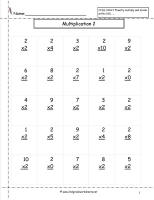Multiplication by Two Facts Worksheet

Common Core State Standards: Extension of CCSS 2.OA.4, CCSS 3.OA.7
Students multiply numbers 2.

###Multiplication by Three With Numberline Worksheet

Common Core State Standards: Extension of CCSS 2.OA.4, CCSS 3.OA.7
Students multiply numbers by 3 with included numberline for skip counting

###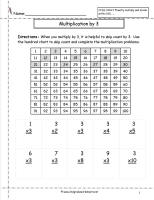Multiplication by Three with Hundred Chart Worksheet

Common Core State Standards: Extension of CCSS 2.OA.4, CCSS 3.OA.7
Students multiply numbers by 3 with included hundred chart for skip counting

###Multiplication by Three Worksheet

Common Core State Standards: Extension of CCSS 2.OA.4, CCSS 3.OA.7
Students multiply numbers by 3.

###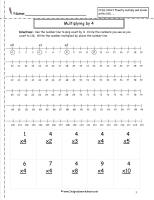Multiplication by Four With Numberline Worksheet

Common Core State Standards: Extension of CCSS 2.OA.4, CCSS 3.OA.7
Students multiply numbers by 4 with included numberline for skip counting

###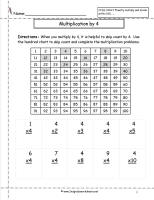Multiplication by Four with Hundred Chart Worksheet

Common Core State Standards: Extension of CCSS 2.OA.4, CCSS 3.OA.7
Students multiply numbers by 4 with included hundred chart for skip counting

###Multiplication by Four Worksheet

Common Core State Standards: Extension of CCSS 2.OA.4, CCSS 3.OA.7
Students multiply numbers by 4.

###Multiplication by Five With Numberline Worksheet

Common Core State Standards: Extension of CCSS 2.OA.4, CCSS 3.OA.7
Students multiply numbers by 5 with included numberline for skip counting

###Multiplication by Five with Hundred Chart Worksheet

Common Core State Standards: Extension of CCSS 2.OA.4, CCSS 3.OA.7
Students multiply numbers by 5 with included hundred chart for skip counting

###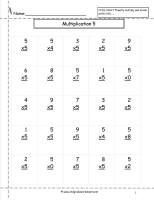Multiplication by Five Worksheet,    2

Common Core State Standards: Extension of CCSS 2.OA.4, CCSS 3.OA.7
Students multiply numbers by 5.

###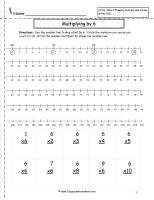Multiplication by Six With Numberline Worksheet

Common Core State Standards: Extension of CCSS 2.OA.4, CCSS 3.OA.7
Students multiply numbers by 6 with included numberline for skip counting

###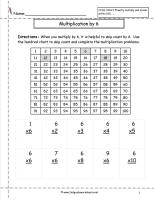Multiplication by Six with Hundred Chart Worksheet

Common Core State Standards: Extension of CCSS 2.OA.4, CCSS 3.OA.7
Students multiply numbers by 6 with included hundred chart for skip counting

###Multiplication by Six Worksheet

Common Core State Standards: Extension of CCSS 2.OA.4, CCSS 3.OA.7
Students multiply numbers by 6.

###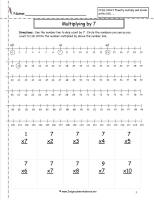Multiplication by Seven With Numberline Worksheet

Common Core State Standards: Extension of CCSS 2.OA.4, CCSS 3.OA.7
Students multiply numbers by 7 with included numberline for skip counting

###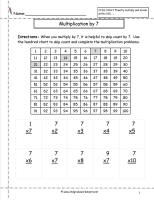Multiplication by Seven with Hundred Chart Worksheet

Common Core State Standards: Extension of CCSS 2.OA.4, CCSS 3.OA.7
Students multiply numbers by 7 with included hundred chart for skip counting

###Multiplication by Seven Worksheet

Common Core State Standards: Extension of CCSS 2.OA.4, CCSS 3.OA.7
Students multiply numbers by 7.

###Multiplication by Eight With Numberline Worksheet

Common Core State Standards: Extension of CCSS 2.OA.4, CCSS 3.OA.7
Students multiply numbers by 8 with included numberline for skip counting### Multiplication by Eight with Hundred Chart Worksheet

Common Core State Standards: Extension of CCSS 2.OA.4, CCSS 3.OA.7
Students multiply numbers by 8 with included hundred chart for skip counting

###Multiplication by Eight Worksheet

Common Core State Standards: Extension of CCSS 2.OA.4, CCSS 3.OA.7
Students multiply numbers by 8.

###Multiplication by Nine With Numberline Worksheet

Common Core State Standards: Extension of CCSS 2.OA.4, CCSS 3.OA.7
Students multiply numbers by 9 with included numberline for skip counting### Multiplication by Nine with Hundred Chart Worksheet

Common Core State Standards: Extension of CCSS 2.OA.4, CCSS 3.OA.7
Students multiply numbers by 9 with included hundred chart for skip counting

###Multiplication by Nine Worksheet

Common Core State Standards: Extension of CCSS 2.OA.4, CCSS 3.OA.7
Students multiply numbers by 9.